# AP Chemistry : Properties of Compounds

## Example Questions

### Example Question #41 : Compounds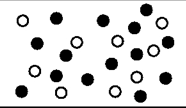Each circle represents an atom and each different color represents a different kind of atom. If two atoms are touching then they are bonded together as a compound.

Which of the following describes the contents of the given image?

Mixture of Compounds

Mixture of Compounds and Elements

Compound

Element

Mixture of Elements

Mixture of Elements

Explanation:

This is a mixture of elements. Each different circle is one type of element. Because there are two different colors and none of them form compounds that means this is a mixture of two different elements.

### Example Question #42 : Compounds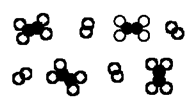Each circle represents an atom and each different color represents a different kind of atom. If two atoms are touching then they are bonded together as a compound.

Which of the following describes the contents of the given image?

Element

Compound

Mixture of Compounds and Elements

Mixture of Compounds

Mixture of Elements

Mixture of Compounds

Explanation:

There are two different molecules in this picture, but because there are no single circles that means this picture is only a mixture of compounds.

### Example Question #43 : Compounds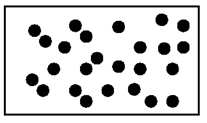Each circle represents an atom and each different color represents a different kind of atom. If two atoms are touching then they are bonded together as a compound.

Which of the following describes the contents of the given image?

Mixture of Compounds and Elements

Mixture of Compounds

Compound

Element

Mixture of Elements

Element

Explanation:

This picture only contains pictures separate circles. All the circles are the same color. Therefore this is a picture of just one element.

### Example Question #44 : Compounds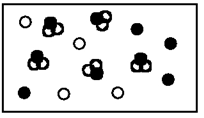Each circle represents an atom and each different color represents a different kind of atom. If two atoms are touching then they are bonded together as a compound.

Which of the following describes the contents of the given image?

Mixture of Elements and Compounds

Compound

Mixture of Elements

Element

Mixture of Compounds

Mixture of Elements and Compounds

Explanation:

This picture contains circles that are two different colors. There is also a molecule. Therefore this is a Mixture of Compounds and Elements.

### Example Question #46 : Compounds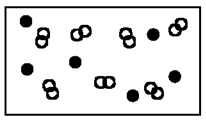Each circle represents an atom and each different color represents a different kind of atom. If two atoms are touching then they are bonded together as a compound.

Which of the following describes the contents of the given image?

Element

Compounds

Mixture of Compounds and Elements

Mixture of Compounds

Mixture of Elements

Mixture of Compounds and Elements

Explanation:

This picture contains one element (single circles) and compounds (joined circles). Therefore this is a Mixture of Compounds and Elements.

### Example Question #47 : Compounds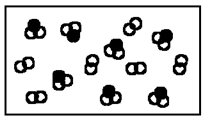Each circle represents an atom and each different color represents a different kind of atom. If two atoms are touching then they are bonded together as a compound.

Which of the following describes the contents of the given image?

Mixture of Elements

Mixture of Compounds and Elements

Element

Mixture of Compounds

Compound

Mixture of Compounds

Explanation:

There are two different types of molecules in this picture (one with two circles, and another with three circles). Therefore this picture is a mixture of compounds.

### Example Question #48 : Compounds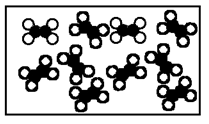Each circle represents an atom and each different color represents a different kind of atom. If two atoms are touching then they are bonded together as a compound.

Which of the following describes the contents of the given image?

Compound

Mixture of Compounds

Mixture of Compounds and Elements

Mixture of Elements

Element

Compound

Explanation:

Although it can hard to to tell, this picture just contains several of the same type of molecules. Therefore this picture is just one compound.

### Example Question #41 : Compounds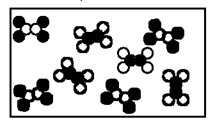Each circle represents an atom and each different color represents a different kind of atom. If two atoms are touching then they are bonded together as a compound.

Which of the following describes the contents of the given image?

Compound

Element

Mixture of Compounds and elements

Mixture of Compounds

Mixture of Elements

Mixture of Compounds

Explanation:

This picture contains two different molecules. One with two black circles and four white circles, and another with two white circles and four black circles. Therefore this picture is a mixture of two compounds.

### Example Question #41 : Compounds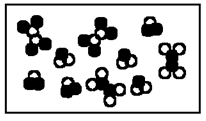Each circle represents an atom and each different color represents a different kind of atom. If two atoms are touching then they are bonded together as a compound.

Which of the following describes the contents of the given image?

Compound

Element

Mixture of Elements

Mixture of Compounds and Elements

Mixture of Compounds

Mixture of Compounds

Explanation:

This picture contains 3 different types of molecules, but no single elements. Therefore this picture is a mixture of compounds.

### Example Question #51 : Compounds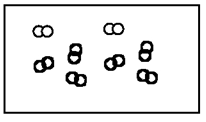Each circle represents an atom and each different color represents a different kind of atom. If two atoms are touching then they are bonded together as a compound.

Which of the following describes the contents of the given image?

Mixture of Elements

Element

Mixture of Compounds

Compound

Mixture of Compounds and Elements